← All Articles

# Writing CNN from Scratch

— Written by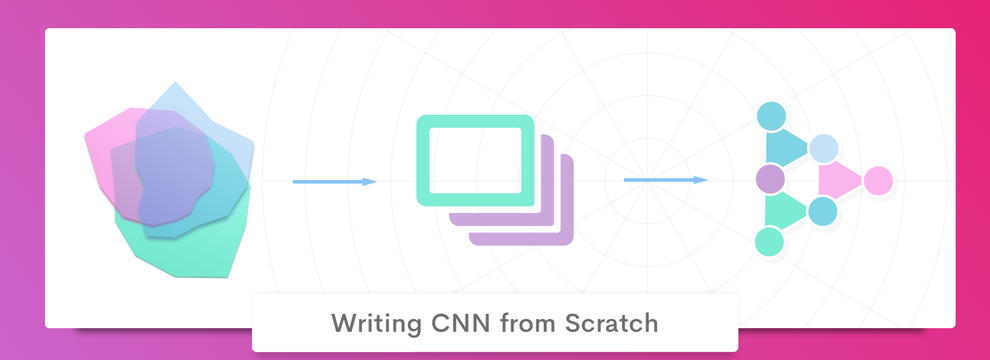Our AI Developer tells you how to get started with Convolutional Neural Networks (CNN) and get beginners’ view on the topic

Before going deep into the technical explanation of CNN, let us understand the necessity of moving from a normal neural network to CNN in the case of any image recognition.

So, what is special about CNN? What fails to work with normal neural network in image recognition, CNN overcomes that failure.

Consider this example. We are training our neural network to find whether the image is of a horse or not. Let’s call it “Horse or Worse” 😋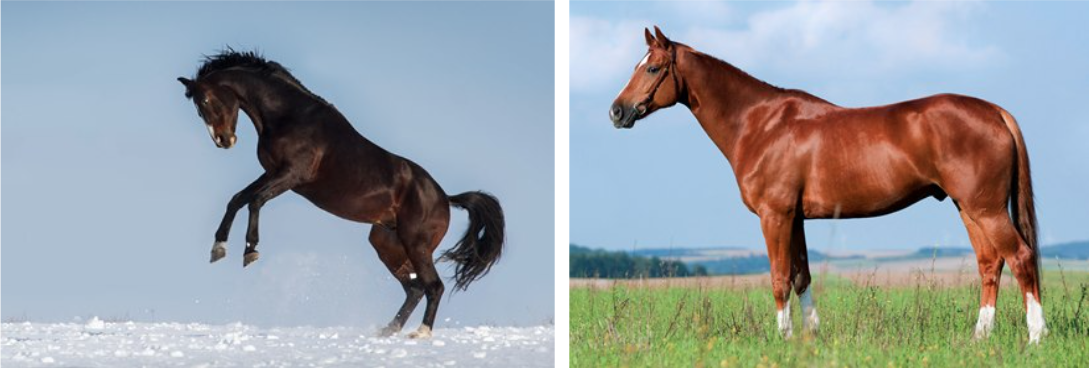Considering a neural network trained with horses facing to the left side,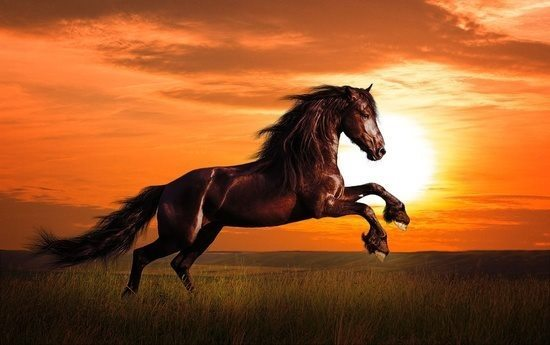So when our network is fed with an image of a horse that faces towards the right side, then it cannot find the horse. That’s bad - then there’s no need to use image recognition. And another limitation is that, if you train the network with the image of a horse in the center of the frame, and if you feed the network with another image where the horse is at the corner of the frame, then it fails to recognize the horse - that’s too bad!

Here, our convolutional neural network comes to rescue us. So what exactly CNN does is that, it removes the limitation of space-oriented recognition.

Now, let’s dive into the technical explanation,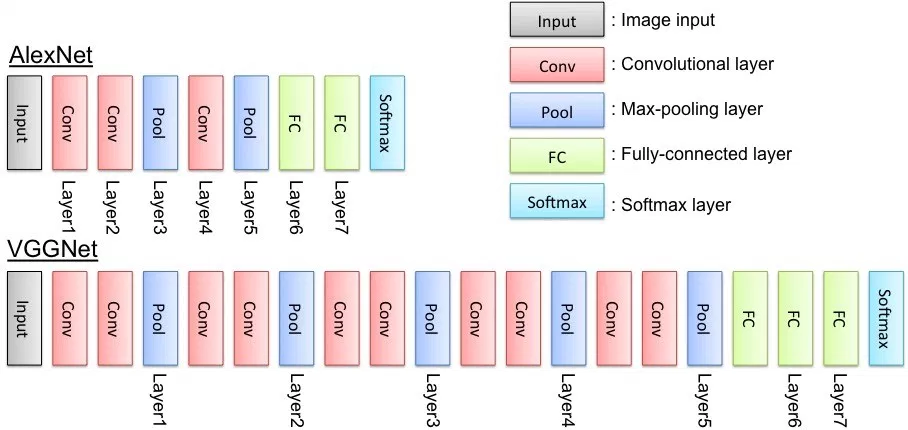Convolutional Layer

Simple Mathematical Explanation on Convolution process.

``````| 5 | 6 | 3 | 1 |
| - | - | - | - |
| 8 | 2 | 1 | 4 |
| 2 | 0 | 1 | 2 |
| 3 | 5 | 1 | 0 |
``````

4x4 Grid - Consider this as the image and the values are pixels (color values)

Choosing the kernel to convolute

Our Kernel size is 2x2. You can find more kernels in this site

Or try experimenting with your own custom kernels 😉

``````| 0 | 1 |
| - | - |
| 0 | 0 |
``````

The image grid and kernels are superimposed and moved over strides. Resulting in a new resized or samesized image based on the stride size. If you move over higher number of strides, ie. take bigger jumps it will result in a smaller sized image.

MaxPooling layer

So what to do after convoluting? Maxpooling! This helps to take out the needed details from the image and gives us lesser number of data points to work out our complex operations on. In technical aspects maxpooling extracts the intensified details that is the resultant of kernel imposing.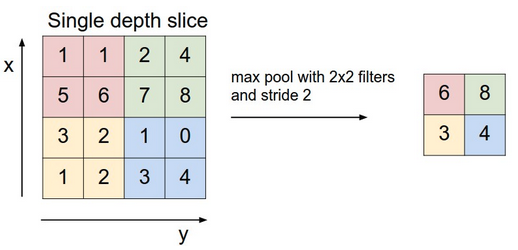For people who can’t understand what a 2x2 filter and stride 2 in image represents, please read the below explanation. Else you can jump into the next layer.

2x2 filter means that, we are considering 2x2 matrix of pixels and stride 2 means that we move 2 columns while moving through y axis and 2 rows while moving through x axis.

Still can’t understand? Ping me!

The Standard way to define the layers is to enclose them inside a function. So that one can make many number of layers. Complex structure can be made easier.

``````def new_weights(shape):
return tf.Variable(tf.truncated_normal(shape, stddev=0.1))

def new_biases(length):
return tf.Variable(tf.constant(0.05, shape=[length]))
def convolution_layer(input, num_of_input_channels, filter_size, num_of_filters, pooling=True):
shape = [filter_size, filter_size,  num_of_input_channels, num_of_filters]
weights = new_weights(shape=shape)
biases = new_biases(length=num_of_filters)
layer = tf.nn.conv2d(input=input, filter=weights, strides=[1, 1, 1, 1], padding='SAME')
layer += biases
if pooling:
layer = max_pooling_layer(layer)
layer = tf.nn.relu(layer)
return layer, weights
def max_pooling_layer(layer, ksize=[1, 2, 2, 1], strides=[1, 2, 2, 1], padding='SAME'):
``````

Fully Connected Layer

This is the final layer where the maxpooled matrix is flattened and even might be resized further. Fully connected layer is later fed into softmax layer in mostcases and the class with higher probability is the prediction.

``````def fully_connected_layer(input, num_inputs, num_outputs, relu=True):
weights = new_weights(shape=[num_inputs, num_outputs])
biases = new_biases(length=num_outputs)

layer = tf.matmul(input, weights) + biases
if relu:
layer = tf.nn.relu(layer)

return layer
``````

This is a simple convolutional network. In CNN, higher the complexity, higher the accuracy. Here’s a link to help you get started with CNN, this link covers all the basics for an introduction into CNN and its useful for beginners, so read up and get started!

Up next

The other side of empowermentSkcript /svr/writing-cnn-from-scratch/ /svrmedia/heroes/cnn.png